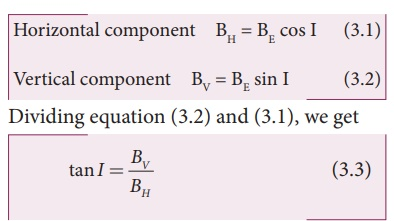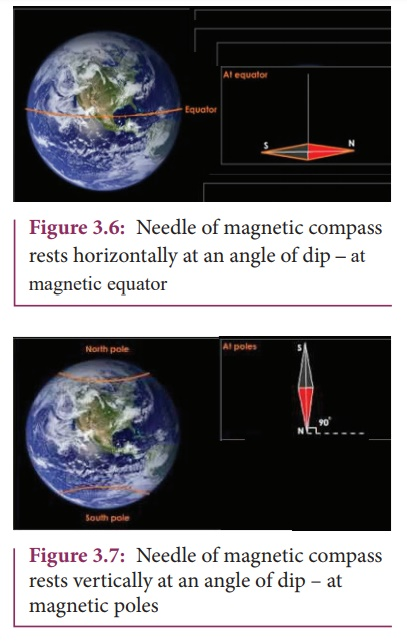Home | | Physics 12th Std | Earth’s magnetic field and magnetic elements

# Earth’s magnetic field and magnetic elements

Gover suggested that the Earth’s magnetic field is due to hot rays coming out from the Sun.

Earth’s magnetic field and magnetic elements

From the activities performed in lower classes, we have noticed that the needle in a magnetic compass or freely suspended magnet comes to rest in a position which is approximately along the geographical north-south direction of the Earth. William Gilbert in 1600 proposed that Earth itself behaves like a gigantic powerful bar magnet.But this theory is not successful because the temperature inside the Earth is very high and so it will not be possible for a magnet to retain its magnetism.

Gover suggested that the Earth’s magnetic field is due to hot rays coming out from the Sun. These rays will heat up the air near equatorial region. Once air becomes hotter, it rises above and will move towards northern and southern hemispheres and get electrified. This may be responsible to magnetize the ferromagnetic materials near the Earth’s surface. Till date, so many theories have been proposed. But none of the theory completely explains the cause for the Earth’s magnetism.

The north pole of magnetic compass needle is attracted towards the magnetic south pole of the Earth which is near the geographic north pole (Figure 3.3). Similarly, the south pole of magnetic compass needle is attracted towards the geographic north pole of the Earth which is near magnetic north-pole. The branch of physics which deals with the Earth’s magnetic field is called Geomagnetism or Terrestrial magnetism.There are three quantities required to specify the magnetic field of the Earth on its surface, which are often called as the elements of the Earth’s magnetic field. They are

(a) magnetic declination (D)

(b) magnetic dip or inclination (I)

(c) the horizontal component of the Earth’s magnetic field (BH)Day and night occur because Earth spins about an axis called geographic axis. A vertical plane passing through the geographic axis is called geographic meridian and a great circle perpendicular to Earth’s geographic axis is called geographic equator.

The straight line which connects magnetic poles of Earth is known as magnetic axis. A vertical plane passing through magnetic axis is called magnetic meridian and a great circle perpendicular to Earth’s magnetic axis is called magnetic equator.

When a magnetic needle is freely suspended, the alignment of the magnet does not exactly lie along the geographic meridian as shown in Figure 3.4. The angle between magnetic meridian at a point and geographical meridian is called the declination or magnetic declination (D). At higher latitudes, the declination is greater whereas near the equator, the declination is smaller. In India, declination angle is very small and for Chennai, magnetic declination angle is -1º 8’ (which is negative (west)).The angle subtended by the Earth’s total magnetic fieldwith the horizontal direction in the magnetic meridian is called dip or magnetic inclination (I) at that point (Figure 3.5). For Chennai, inclination angle is 14o 16’. The component of Earth’s magnetic field along the horizontal direction in the magnetic meridian is called horizontal component of Earth’s magnetic field, denoted by BH.

Let BE be the net Earth’s magnetic field at a point P on the surface of the Earth. BE can be resolved into two perpendicular components.## (i) At magnetic equator

The Earth’s magnetic field is parallel to the surface of the Earth (i.e., horizontal) which implies that the needle of magnetic compass rests horizontally at an angle of dip, I = 0o as shown in figure 3.6.

BH = BE

BV = 0

This implies that the horizontal component is maximum at equator and vertical component is zero at equator.

## (ii) At magnetic poles

The Earth’s magnetic field is perpendicular to the surface of the Earth (i.e., vertical) which implies that the needle of magnetic compass rests vertically at an angle of dip, I = 90º as shown in Figure 3.7. Hence,BH = 0

BV = BE

This implies that the vertical component is maximum at poles and horizontal component is zero at poles.

EXAMPLE 3.1

The horizontal component and vertical components of Earth’s magnetic field at a place are 0.15 G and 0.26 G respectively. Calculate the angle of dip and resultant magnetic field.

Solution:

BH = 0.15 G and BV = 0.26 G

tan I = 00.26/0.15  I = tan −1(1. 732) =60º

The resultant magnetic field of the Earth isStudy Material, Lecturing Notes, Assignment, Reference, Wiki description explanation, brief detail
12th Physics : Magnetism and Magnetic Effects of Electric Current : Earth’s magnetic field and magnetic elements |